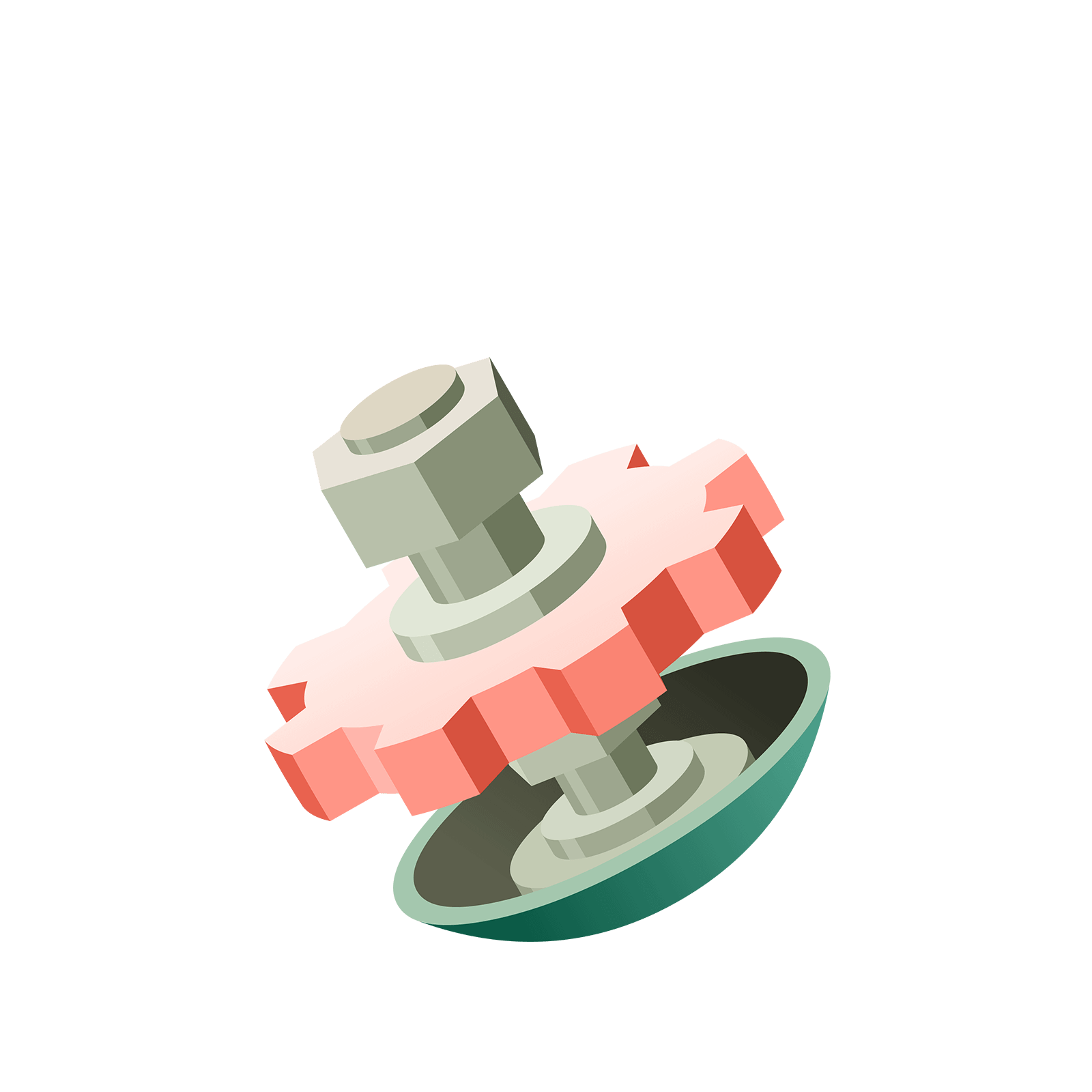05 217 385# Station  BWild ExplorationHere are some investigations you might want to explore, or at least think about.
Everything will become clear as we learn more in the next chapters, but you might find it fun to mull on these “big questions” ideas right away.

# EXPLORATION 1: What are these machines actually doing?

Can you figure out what these machines are actually doing?
Why, in a $1\leftarrow 10$ machine, is the code for two hundred and seventy three "$273$"?
Are all the codes for numbers in a $1\leftarrow 10$ sure to be identical to how we normally write numbers?

If we can answer this question for the $1\leftarrow 10$ machine, then can we make sense of all the codes for a $1\leftarrow 2$ machine? What does the code $1101$ for the number thirteen mean?

Comment: Of course, we will answer these questions in the next island, Insighto, but it might be rewarding to think about these ideas questions on your own first!

# Does the order in which one explodes dots seem to matter?

Here are $19$ dots loaded into the rightmost box of a $1 \leftarrow 2$ machine.
Try exploding pairs of dots in a haphazard manner: explode a few pairs from the rightmost box, then some from the second box and then some more back from the first box, and some more from the second box, and so on, until you reach a stable code. Then do it again!

Does the same final code of $10011$ seem to appear each and every time you do this?You can either play with some of the optional stations below or go to the next island!